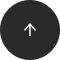MySQL

# How to Calculate a Square in SQL

POWER

#### Problem:

You want to find the square of a number in SQL Server.

#### Example:

You want to compute the square of each number in the column `number` from the table `data`.

number
3
1
0.5
0
-2

#### Solution 1:

```SELECT
number,
SQUARE(number) AS square
FROM data;
```

#### Solution 2:

```SELECT
number,
number * number AS square
FROM data;
```

#### Solution 3:

```SELECT
number,
POWER(number, 2) AS square
FROM data;
```

The result is:

numbersquare
39
11
0.50.25
00
-24

#### Discussion:

One way to compute the square of a number in SQL Server is to use the `SQUARE()` function. It takes a number as an argument and returns the squared number.

The square of a number can also be computed as `number * number`, so another way is to simply use this expression; no additional function is needed.

The third way to compute the square of a number is to use the `POWER()` function. This function takes a `number` and a `power` as arguments and returns the powered number. Here, you need to compute the square, so the `power` is 2. So, you have `POWER(number, 2)`.

Similarly, you can calculate any power of a number, e.g. the third power.

```SELECT
POWER(number, 3) AS third_power
FROM data;
```

The result will be:

numberthird_power
327
11
0.50.125
00
-2-8

#### Recommended articles: